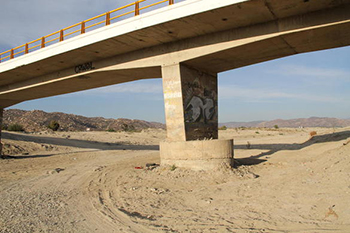online_scour_froehlich1989:  Calculation of abutment scour using the Froehlich 1989 equationFormula:

Fr = v /(gya)1/2

(ys / ya) = 2.27 K1 K2 (L' / ya)0.43 Fr 0.61 + 1

[ SI Units (metric):  ys, ya and L' in m ]
[ U.S. Customary Units:  ys, ya and L' in ft ]
[ Fr = Froude number; g = gravitational acceleration ]

Reference:

 SAMPLE INPUT      [Main page]     [Description] Flow depth directly upstream of the pier y1 =  1.0 m Mean flow velocity directly upstream of the pier v1 =  1.0 m/s Length of flow obstructed by the embankment L' =  5.0 m Angle of embankment with the flow θ =  90 degrees Abutment shape :  Vertical wall SAMPLE OUTPUT               Scour depth ys =   3.26 m